Register for Maths, Science, English, Reasoning Olympiad Exams Register here | Book Free Demo for Live Olympiad Classes here | Check Olympiad Exam Dates here | Buy Practice Papers for IMO, IOM, HEO, IOEL etc here | Login here to participate in all India free mock test on every Saturday

# Subtraction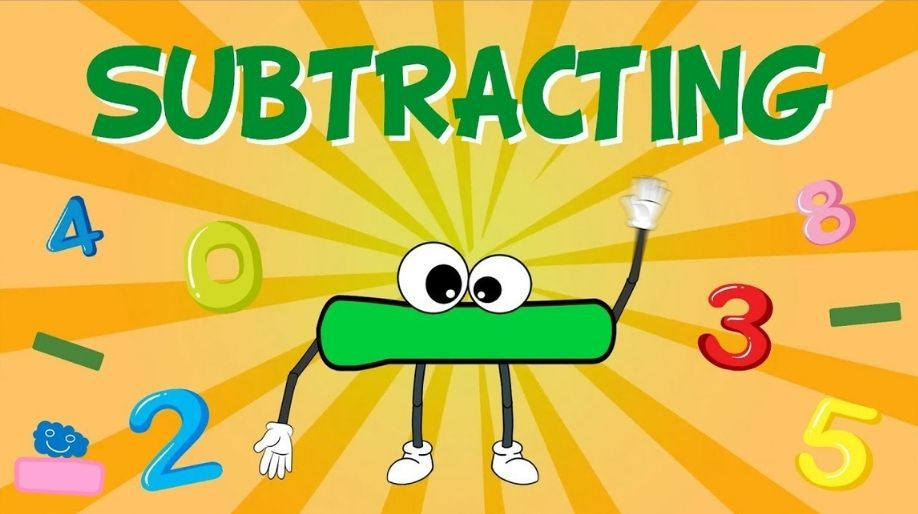What is subtraction? Subtraction is the process of taking away something from total amount or value and the left part is always less than total value. Do you want to know if you have 20 Rupees and you eat chocolate for 5 Rupees then with how much rupees are you left with? To know this, study Subtraction with me.

Know Subtraction with Fun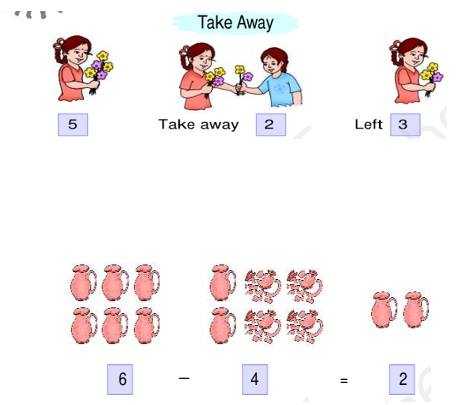Do you want to know Different terms related to Subtraction? Come and learn with me.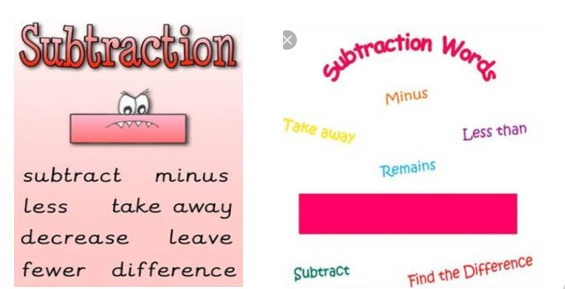Let us learn simple strategies and rules to do Subtraction to easily know amount left with you after giving some amount to your friend.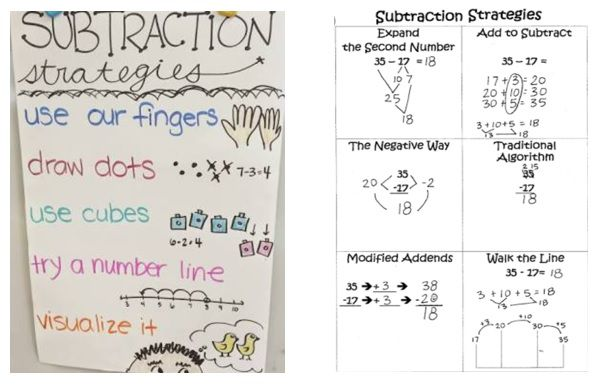Solved examples of subtraction

Examples of subtraction of single digit number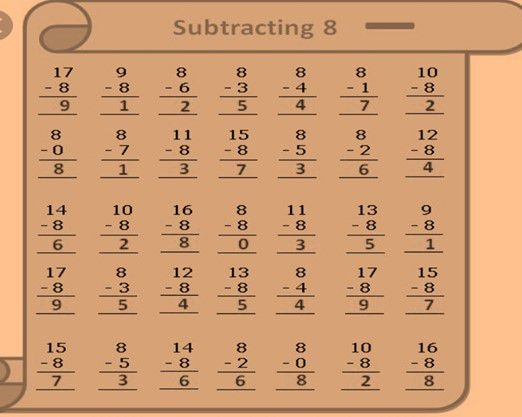Examples of subtraction of three-digit numbers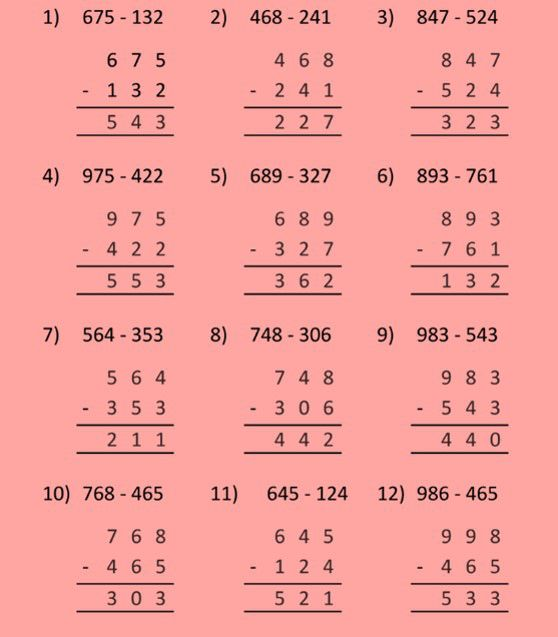Examples on subtraction of four digit numbers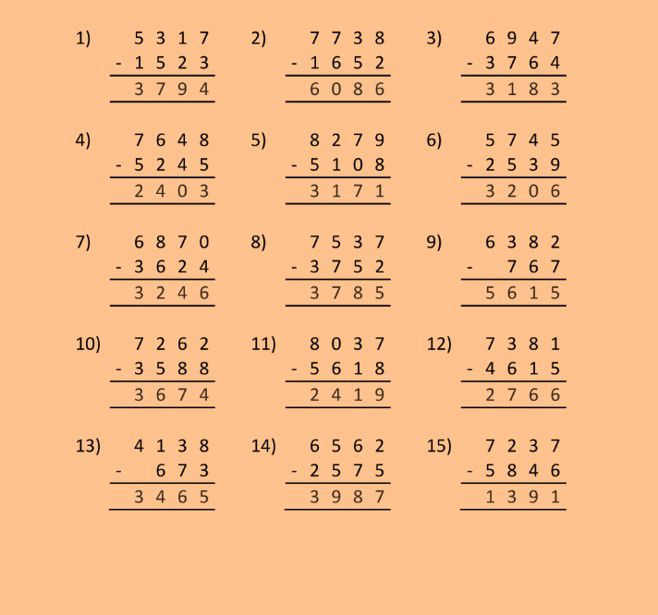Practice Questions on Subtraction

Practice Questions on single digit numbers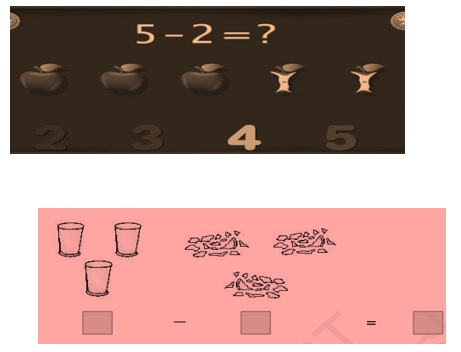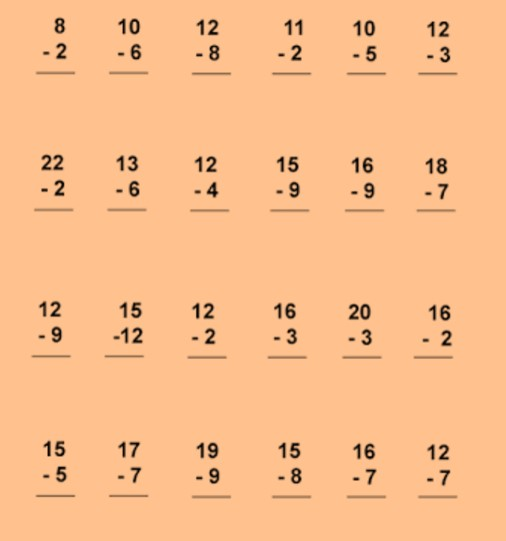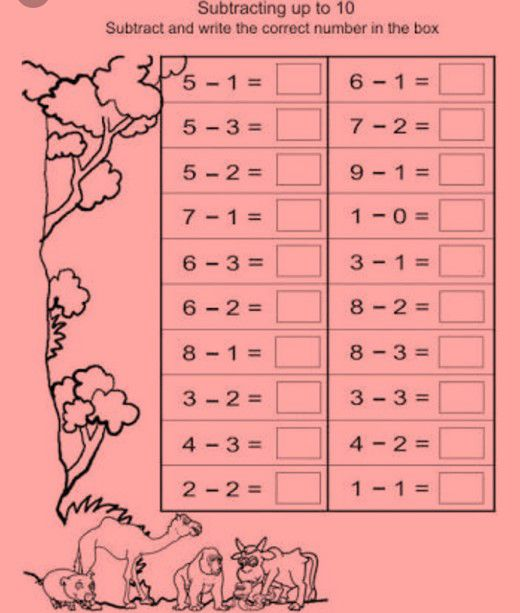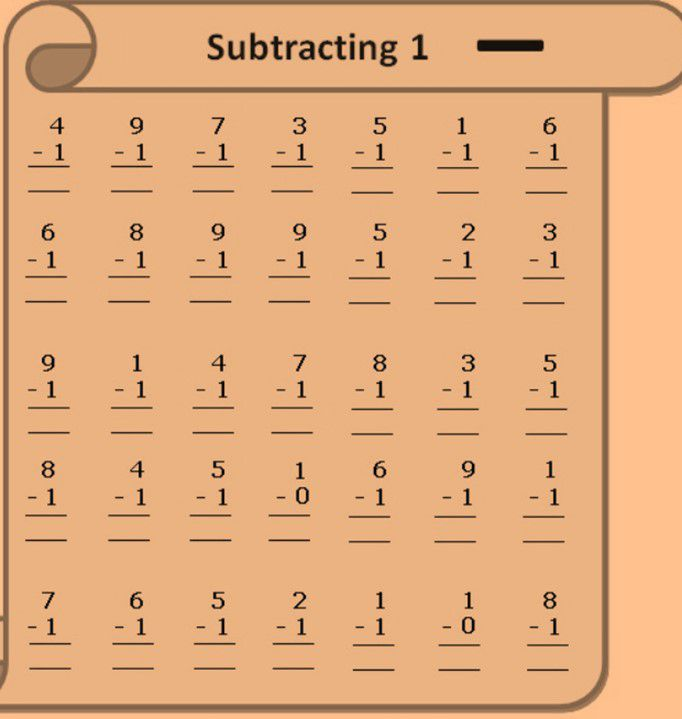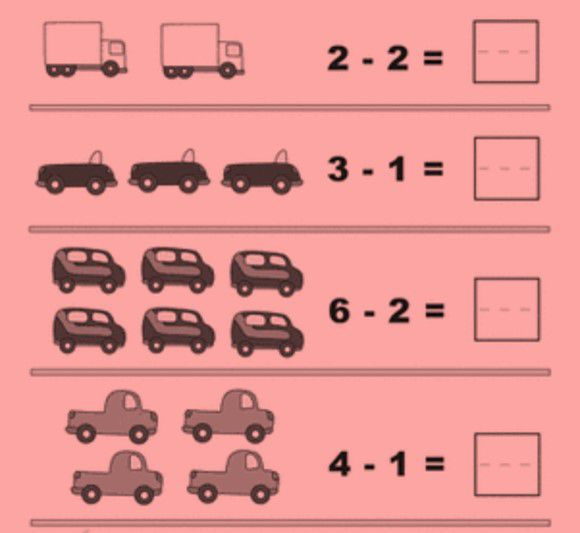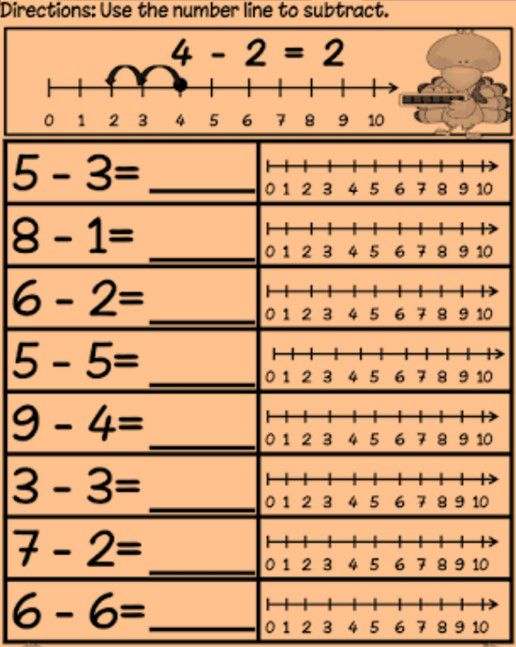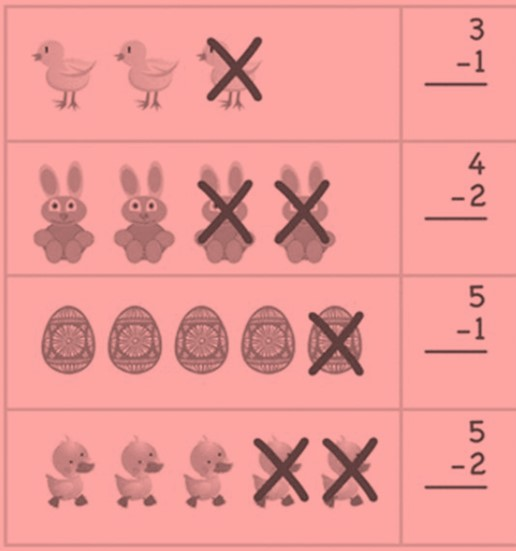Work out missing Subtraction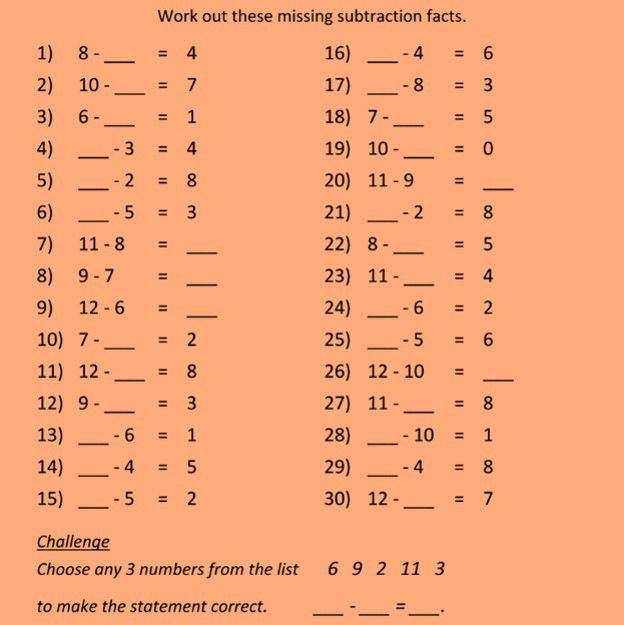Practice Questions on three digit numbers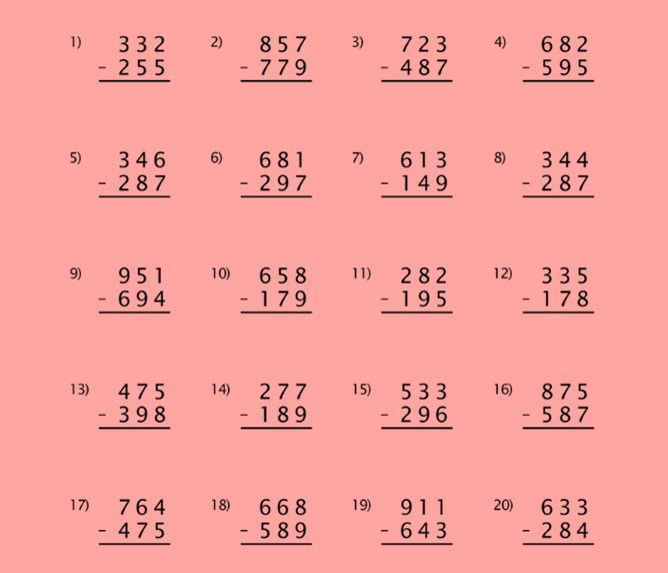Recap

• Subtraction is the process of finding left portion out of total thing.
• In subtraction the left part is always less than total quantity.
• Subtraction is also known as minus, difference, take away and left.## Quiz for Subtraction

 Q.1 How many balls are left?a) 11 b) 10 c) 9 d) 8
 Q.2 Sonali has 17 crayons. Rekha has 2 fewer crayons than Sonali. How many crayons does Rekha have? a) 19 b) 14 c) 15 d) 16
 Q.3 How many more cars are there than airplane?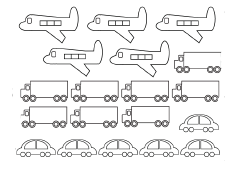a) 1 b) 2 c) 3 d) 4
 Q.4 Calculate the number of gifts left.a) 2 b) 3 c) 4 d) 5
 Q.5 50 pears are in the basket. 21 are red and the rest are green. How many pears are green? a) 27 b) 28 c) 29 d) 30
 Q.6 How many houses are left?a) 3 b) 4 c) 5 d) 6
 Q.7 Aditi found 5 tomatoes on her plant. She left one and took all others for making salad. How many tomatoes did she use for making the salad? a) 1 b) 2 c) 3 d) 4
 Q.8 Chintu borrowed Rs.45 from Amit. If Amit had Rs.89, how much does he have now? a) Rs. 41 b) Rs. 42 c) Rs. 43 d) Rs. 44
 Q.9 Calculate the number of lollipops left.a) 1 b) 2 c) 3 d) 4
 Q.10 This creature had 8 teeth. How many did it lose?a) 3 b) 4 c) 5 d) 6